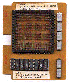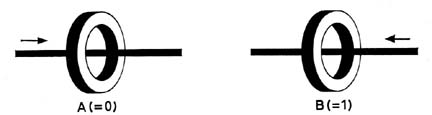Magnetic Core Memory'sSection Three - The Rectangular Hysteresis Loop

## The Rectangular Hysteresis Loop

So far we have discussed, in a very simple way, the principles on which a magnetic memory is based. It is now necessary to consider certain aspects of the subject from a more technical angle, and in particular to explain more fully why the material 'ferroxcube 6' has been selected for use in the equipment to be described.Fig4

It has been shown that the direction of the magnetisation of a particular ring element is determined by the direction of the current flowing through the wire on which the ring is threaded (see Fig. 4). It should also be understood that the extent to which a ring is magnetised is proportional to the current flowing through the wire, in other words to the Strength of the magnetising field.

The relationship between the direction and strength of the resultant magnetisation (B). and the direction and strength of the magnetising force(H), for the material ferroxcube 6 is shown once more in the graphs reproduced in Fig. 5a.Fig 5a

Depending upon what has previously happened by way of applying a magnetising force H, a ring core, when no current is flowing in the wire threaded through it, will, due to the special form of the hysteresis loop, be in one of two magnetic conditions: it will either be - magnetised in the direction we will call '+', to an extent +B, or in the direction we will call '--' to the extent -B, The condition +B, is used to represent the symbol '1', and the condition -B,. to represent the symbol 0. In order to change the magnetic condition of the ring core from +Br to -B, or vice versa, a current corresponding to a magnetising force H must pass through the wire. In actual practice, where a number of wires pass through each ring core, the algebraic sum of the currents in all the wires must be equivalent to a magnetising force H.

For example,. imagine that a particular ring is in the condition +B, representing the symbol 1. If a current equivalent to a field of -H is now passed through the wire (see lowest horizontal block in Fig. 5b), the magnetisation will be reversed., the condition changing to that corresponding to point P on the graph. When the current ceases (H becoming zero) the strength of the magnetisation will fall to the value marked -B, that is to say the ring will be in the condition representing the symbol 0.Fig5b

Similarly, if the ring core is originally in the condition -B, the application of a current pulse corresponding to a magnetising force of +H will change the magnetisation to the point Q on the graph and, on cessation of the current, the magnetisation. will drop to the value +Br.

Again, because of the rectangular form of the hysteresis loop, the application of a current pulse corresponding. to a magnetising force of 1/2 H in either direction will make no permanent change in the direction of the magnetisation. If the core is in the condition +Br, the application of a magnetising field of 1/2 H will temporarily drive the magnetisation either to the value represented by +R on the graph or to the value represented by -R, and on cessation of the current the magnetisation will return to the value +Br.

Similarly, if the core is in the condition -B, the application of a magnetising field of 1H will temporarily drive the magnetisation either to the value represented by -S or to the value represented by -S1. On cessation of the current the magnetisation will again return to -Br.

Furthermore, the graph shows that if the core is in the condition +B,. and a current pulse corresponding to a field of +H is applied, the magnetism will not change permanently, but will merely rise to the value represented by point Q, returning to the value +B,. when the current ceases. Similarly, if the core is in the condition -B, the application of a field of value -H will merely temporarily increase the magnetisation to a value represented by point P, and on cessation of the current the magnetisation will again become -B,

The above effects are due to the rectangular form of the hysteresis loop, of the special grade of ferroxcube known as 'ferroxcube W.